# _Draft__Asset_Supply_and_Liquidity_Transformation_in_HANK (2).pdf

24. Jan 2023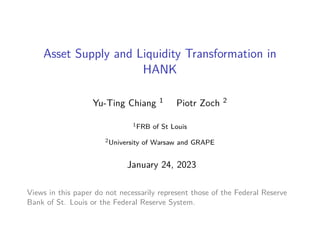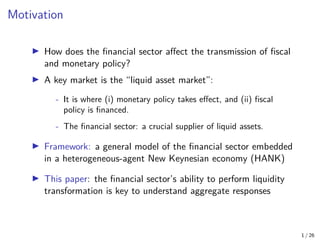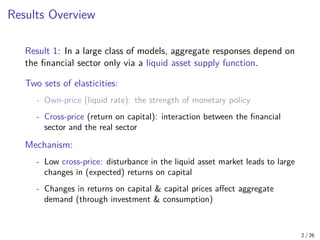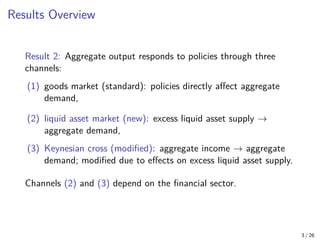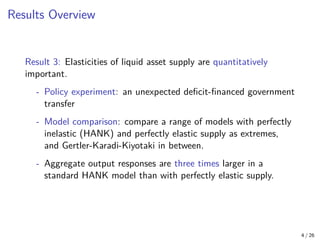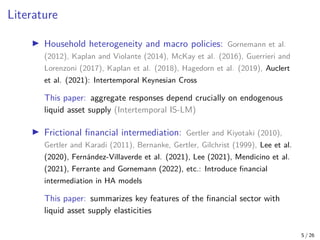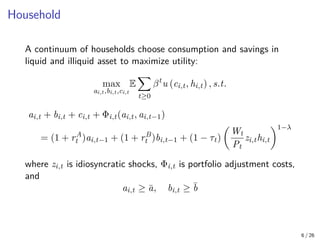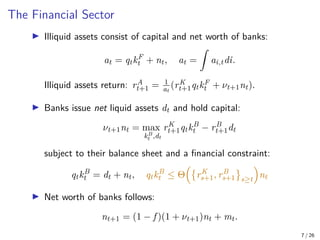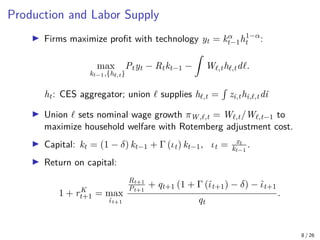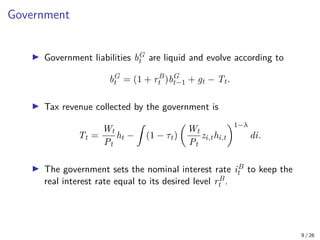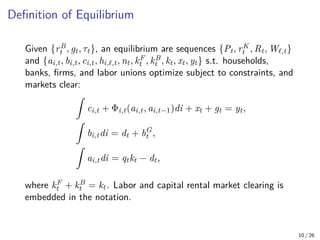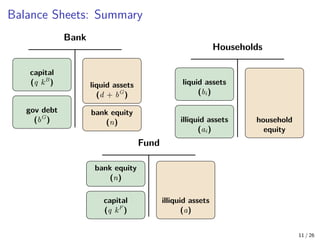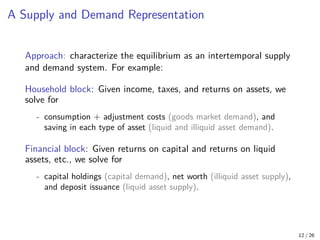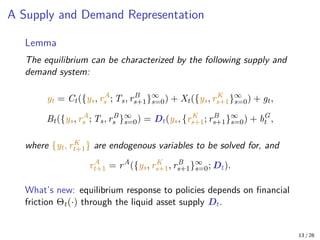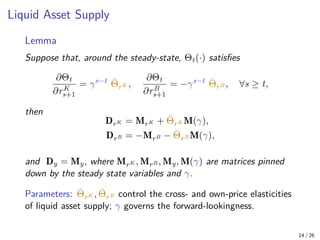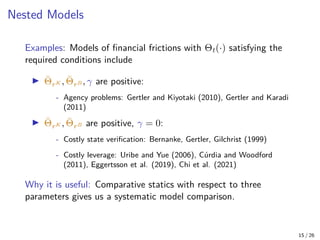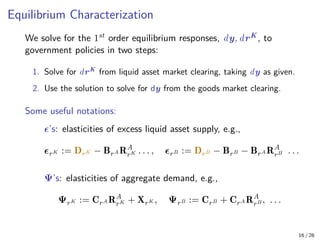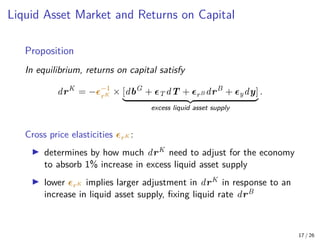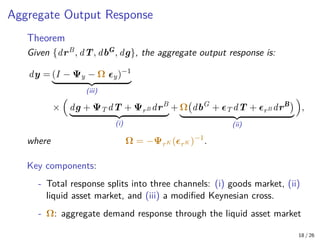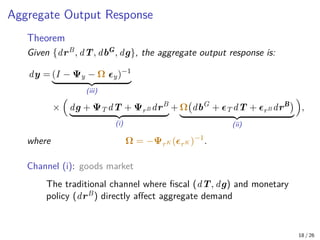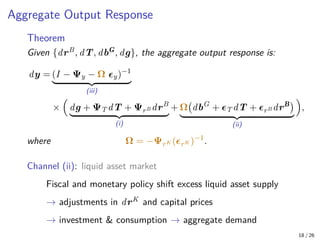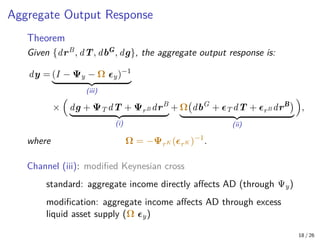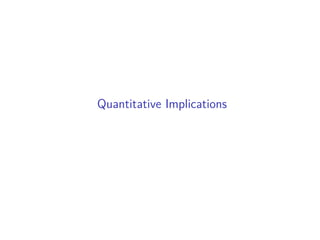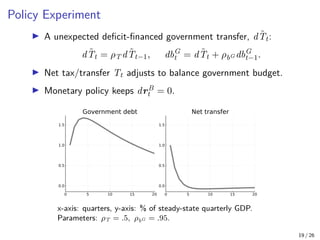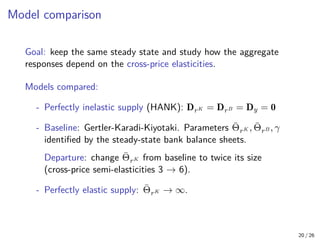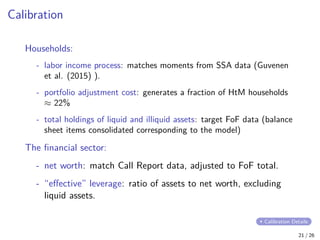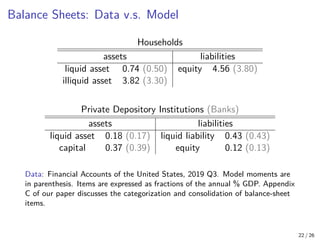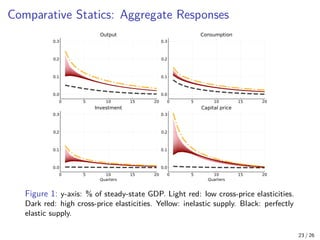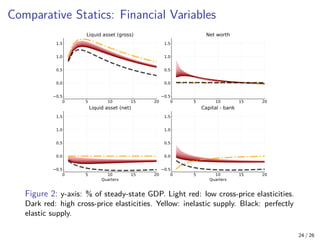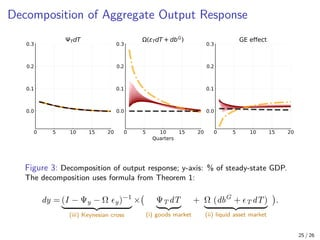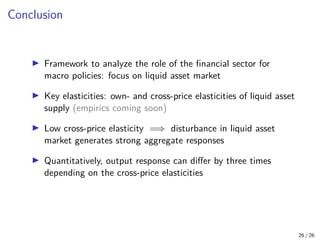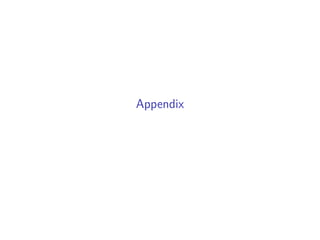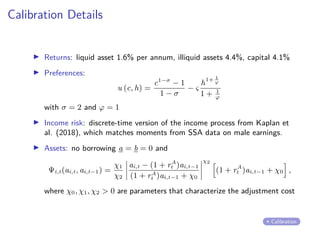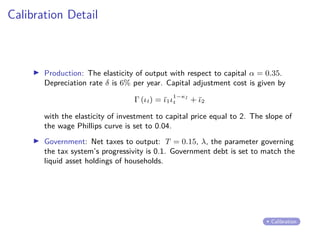1 von 36

### _Draft__Asset_Supply_and_Liquidity_Transformation_in_HANK (2).pdf

• 1. Asset Supply and Liquidity Transformation in HANK Yu-Ting Chiang 1 Piotr Zoch 2 1FRB of St Louis 2University of Warsaw and GRAPE January 24, 2023 Views in this paper do not necessarily represent those of the Federal Reserve Bank of St. Louis or the Federal Reserve System.
• 2. Motivation I How does the financial sector affect the transmission of fiscal and monetary policy? I A key market is the “liquid asset market”: - It is where (i) monetary policy takes effect, and (ii) fiscal policy is financed. - The financial sector: a crucial supplier of liquid assets. I Framework: a general model of the financial sector embedded in a heterogeneous-agent New Keynesian economy (HANK) I This paper: the financial sector’s ability to perform liquidity transformation is key to understand aggregate responses 1 / 26
• 3. Results Overview Result 1: In a large class of models, aggregate responses depend on the financial sector only via a liquid asset supply function. Two sets of elasticities: - Own-price (liquid rate): the strength of monetary policy - Cross-price (return on capital): interaction between the financial sector and the real sector Mechanism: - Low cross-price: disturbance in the liquid asset market leads to large changes in (expected) returns on capital - Changes in returns on capital & capital prices affect aggregate demand (through investment & consumption) 2 / 26
• 4. Results Overview Result 2: Aggregate output responds to policies through three channels: (1) goods market (standard): policies directly affect aggregate demand, (2) liquid asset market (new): excess liquid asset supply → aggregate demand, (3) Keynesian cross (modified): aggregate income → aggregate demand; modified due to effects on excess liquid asset supply. Channels (2) and (3) depend on the financial sector. 3 / 26
• 5. Results Overview Result 3: Elasticities of liquid asset supply are quantitatively important. - Policy experiment: an unexpected deficit-financed government transfer - Model comparison: compare a range of models with perfectly inelastic (HANK) and perfectly elastic supply as extremes, and Gertler-Karadi-Kiyotaki in between. - Aggregate output responses are three times larger in a standard HANK model than with perfectly elastic supply. 4 / 26
• 6. Literature I Household heterogeneity and macro policies: Gornemann et al. (2012), Kaplan and Violante (2014), McKay et al. (2016), Guerrieri and Lorenzoni (2017), Kaplan et al. (2018), Hagedorn et al. (2019), Auclert et al. (2021): Intertemporal Keynesian Cross This paper: aggregate responses depend crucially on endogenous liquid asset supply (Intertemporal IS-LM) I Frictional financial intermediation: Gertler and Kiyotaki (2010), Gertler and Karadi (2011), Bernanke, Gertler, Gilchrist (1999), Lee et al. (2020), Fernández-Villaverde et al. (2021), Lee (2021), Mendicino et al. (2021), Ferrante and Gornemann (2022), etc.: Introduce financial intermediation in HA models This paper: summarizes key features of the financial sector with liquid asset supply elasticities 5 / 26
• 8. Household A continuum of households choose consumption and savings in liquid and illiquid asset to maximize utility: max ai,t,bi,t,ci,t E X t≥0 βt u (ci,t, hi,t) , s.t. ai,t + bi,t + ci,t + Φi,t(ai,t, ai,t−1) = (1 + rA t )ai,t−1 + (1 + rB t )bi,t−1 + (1 − τt) Wt Pt zi,thi,t 1−λ where zi,t is idiosyncratic shocks, Φi,t is portfolio adjustment costs, and ai,t ≥ ā, bi,t ≥ b̄ 6 / 26
• 9. The Financial Sector I Illiquid assets consist of capital and net worth of banks: at = qtkF t + nt, at = Z ai,tdi. Illiquid assets return: rA t+1 = 1 at (rK t+1qtkF t + νt+1nt). I Banks issue net liquid assets dt and hold capital: νt+1nt = max kB t ,dt rK t+1qtkB t − rB t+1dt subject to their balance sheet and a financial constraint: qtkB t = dt + nt, qtkB t ≤ Θ rK s+1, rB s+1 s≥t nt I Net worth of banks follows: nt+1 = (1 − f )(1 + νt+1)nt + mt. 7 / 26
• 10. Production and Labor Supply I Firms maximize profit with technology yt = kα t−1h1−α t : max kt−1,{h,t} Ptyt − Rtkt−1 − Z W,th,td. ht: CES aggregator; union  supplies h,t = R zi,thi,,tdi I Union  sets nominal wage growth πW,,t = W,t/W,t−1 to maximize household welfare with Rotemberg adjustment cost. I Capital: kt = (1 − δ) kt−1 + Γ (ιt) kt−1, ιt = xt kt−1 . I Return on capital: 1 + rK t+1 = max ι̂t+1 Rt+1 Pt+1 + qt+1 (1 + Γ (ι̂t+1) − δ) − ι̂t+1 qt . 8 / 26
• 11. Government I Government liabilities bG t are liquid and evolve according to bG t = (1 + rB t )bG t−1 + gt − Tt. I Tax revenue collected by the government is Tt = Wt Pt ht − Z (1 − τt) Wt Pt zi,thi,t 1−λ di. I The government sets the nominal interest rate iB t to keep the real interest rate equal to its desired level rB t . 9 / 26
• 12. Definition of Equilibrium Given {rB t , gt, τt}, an equilibrium are sequences {Pt, rK t , Rt, W,t} and {ai,t, bi,t, ci,t, hi,`,t, nt, kF t , kB t , kt, xt, yt} s.t. households, banks, firms, and labor unions optimize subject to constraints, and markets clear: Z ci,t + Φi,t(ai,t, ai,t−1)di + xt + gt = yt, Z bi,tdi = dt + bG t , Z ai,tdi = qtkt − dt, where kF t + kB t = kt. Labor and capital rental market clearing is embedded in the notation. 10 / 26
• 13. Balance Sheets: Summary Households liquid assets (bi) illiquid assets (ai) household equity Bank capital (q kB ) gov debt (bG ) liquid assets (d + bG ) bank equity (n) Fund bank equity (n) capital (q kF ) illiquid assets (a) 11 / 26
• 14. Aggregate Responses with Financial Frictions
• 15. A Supply and Demand Representation Approach: characterize the equilibrium as an intertemporal supply and demand system. For example: Household block: Given income, taxes, and returns on assets, we solve for - consumption + adjustment costs (goods market demand), and saving in each type of asset (liquid and illiquid asset demand). Financial block: Given returns on capital and returns on liquid assets, etc., we solve for - capital holdings (capital demand), net worth (illiquid asset supply), and deposit issuance (liquid asset supply). 12 / 26
• 16. A Supply and Demand Representation Lemma The equilibrium can be characterized by the following supply and demand system: yt = Ct({ys, rA s ; Ts, rB s+1}∞ s=0) + Xt({ys, rK s+1}∞ s=0) + gt, Bt({ys, rA s ; Ts, rB s }∞ s=0) = Dt(ys, {rK s+1; rB s+1}∞ s=0) + bG t , where {yt, rK t+1} are endogenous variables to be solved for, and rA t+1 = rA ({ys, rK s+1, rB s+1}∞ s=0; Dt). What’s new: equilibrium response to policies depends on financial friction Θt(·) through the liquid asset supply Dt. 13 / 26
• 17. Liquid Asset Supply Lemma Suppose that, around the steady-state, Θt(·) satisfies ∂Θt ∂rK s+1 = γs−t Θ̄rK , ∂Θt ∂rB s+1 = −γs−t Θ̄rB , ∀s ≥ t, then DrK = MrK + Θ̄rK M(γ), DrB = −MrB − Θ̄rB M(γ), and Dy = My, where MrK , MrB , My, M(γ) are matrices pinned down by the steady state variables and γ. Parameters: Θ̄rK , Θ̄rB control the cross- and own-price elasticities of liquid asset supply; γ governs the forward-lookingness. 14 / 26
• 18. Nested Models Examples: Models of financial frictions with Θt(·) satisfying the required conditions include I Θ̄rK , Θ̄rB , γ are positive: - Agency problems: Gertler and Kiyotaki (2010), Gertler and Karadi (2011) I Θ̄rK , Θ̄rB are positive, γ = 0: - Costly state verification: Bernanke, Gertler, Gilchrist (1999) - Costly leverage: Uribe and Yue (2006), Cúrdia and Woodford (2011), Eggertsson et al. (2019), Chi et al. (2021) Why it is useful: Comparative statics with respect to three parameters gives us a systematic model comparison. 15 / 26
• 19. Equilibrium Characterization We solve for the 1st order equilibrium responses, dy, drK , to government policies in two steps: 1. Solve for drK from liquid asset market clearing, taking dy as given. 2. Use the solution to solve for dy from the goods market clearing. Some useful notations: ’s: elasticities of excess liquid asset supply, e.g., rK := DrK − BrA RA rK . . . , rB := DrB − BrB − BrA RA rB . . . Ψ’s: elasticities of aggregate demand, e.g., ΨrK := CrA RA rK + XrK , ΨrB := CrB + CrA RA rB , . . . 16 / 26
• 20. Liquid Asset Market and Returns on Capital Proposition In equilibrium, returns on capital satisfy drK = −−1 rK × [dbG + T dT + rB drB + ydy] | {z } excess liquid asset supply . Cross price elasticities rK : I determines by how much drK need to adjust for the economy to absorb 1% increase in excess liquid asset supply I lower rK implies larger adjustment in drK in response to an increase in liquid asset supply, fixing liquid rate drB 17 / 26
• 21. Aggregate Output Response 18 / 26 Theorem Given {drB, dT, dbG, dg}, the aggregate output response is: dy = (I − Ψy − Ω y)−1 | {z } (iii) × dg + ΨT dT + ΨrB drB | {z } (i) + Ω dbG + T dT + rB drB | {z } (ii) , where Ω = −ΨrK (rK )−1 . Key components: - Total response splits into three channels: (i) goods market, (ii) liquid asset market, and (iii) a modified Keynesian cross. - Ω: aggregate demand response through the liquid asset market
• 22. Aggregate Output Response 18 / 26 Theorem Given {drB, dT, dbG, dg}, the aggregate output response is: dy = (I − Ψy − Ω y)−1 | {z } (iii) × dg + ΨT dT + ΨrB drB | {z } (i) + Ω dbG + T dT + rB drB | {z } (ii) , where Ω = −ΨrK (rK )−1 . Channel (i): goods market The traditional channel where fiscal (dT, dg) and monetary policy (drB) directly affect aggregate demand
• 23. Aggregate Output Response 18 / 26 Theorem Given {drB, dT, dbG, dg}, the aggregate output response is: dy = (I − Ψy − Ω y)−1 | {z } (iii) × dg + ΨT dT + ΨrB drB | {z } (i) + Ω dbG + T dT + rB drB | {z } (ii) , where Ω = −ΨrK (rK )−1 . Channel (ii): liquid asset market Fiscal and monetary policy shift excess liquid asset supply → adjustments in drK and capital prices → investment consumption → aggregate demand
• 24. Aggregate Output Response 18 / 26 Theorem Given {drB, dT, dbG, dg}, the aggregate output response is: dy = (I − Ψy − Ω y)−1 | {z } (iii) × dg + ΨT dT + ΨrB drB | {z } (i) + Ω dbG + T dT + rB drB | {z } (ii) , where Ω = −ΨrK (rK )−1 . Channel (iii): modified Keynesian cross standard: aggregate income directly affects AD (through Ψy) modification: aggregate income affects AD through excess liquid asset supply (Ω y)
• 26. Policy Experiment I A unexpected deficit-financed government transfer, dT̃t: dT̃t = ρT dT̃t−1, dbG t = dT̃t + ρbG dbG t−1. I Net tax/transfer Tt adjusts to balance government budget. I Monetary policy keeps drB t = 0. x-axis: quarters, y-axis: % of steady-state quarterly GDP. Parameters: ρT = .5, ρbG = .95. 19 / 26
• 27. Model comparison Goal: keep the same steady state and study how the aggregate responses depend on the cross-price elasticities. Models compared: - Perfectly inelastic supply (HANK): DrK = DrB = Dy = 0 - Baseline: Gertler-Karadi-Kiyotaki. Parameters Θ̄rK , Θ̄rB , γ identified by the steady-state bank balance sheets. Departure: change Θ̄rK from baseline to twice its size (cross-price semi-elasticities 3 → 6). - Perfectly elastic supply: Θ̄rK → ∞. 20 / 26
• 28. Calibration Households: - labor income process: matches moments from SSA data (Guvenen et al. (2015) ). - portfolio adjustment cost: generates a fraction of HtM households ≈ 22% - total holdings of liquid and illiquid assets: target FoF data (balance sheet items consolidated corresponding to the model) The financial sector: - net worth: match Call Report data, adjusted to FoF total. - “effective” leverage: ratio of assets to net worth, excluding liquid assets. 21 / 26 Calibration Details
• 29. Balance Sheets: Data v.s. Model Households assets liabilities liquid asset 0.74 (0.50) equity 4.56 (3.80) illiquid asset 3.82 (3.30) Private Depository Institutions (Banks) assets liabilities liquid asset 0.18 (0.17) liquid liability 0.43 (0.43) capital 0.37 (0.39) equity 0.12 (0.13) Data: Financial Accounts of the United States, 2019 Q3. Model moments are in parenthesis. Items are expressed as fractions of the annual % GDP. Appendix C of our paper discusses the categorization and consolidation of balance-sheet items. 22 / 26
• 30. Comparative Statics: Aggregate Responses Figure 1: y-axis: % of steady-state GDP. Light red: low cross-price elasticities. Dark red: high cross-price elasticities. Yellow: inelastic supply. Black: perfectly elastic supply. 23 / 26
• 31. Comparative Statics: Financial Variables Figure 2: y-axis: % of steady-state GDP. Light red: low cross-price elasticities. Dark red: high cross-price elasticities. Yellow: inelastic supply. Black: perfectly elastic supply. 24 / 26
• 32. Decomposition of Aggregate Output Response Figure 3: Decomposition of output response; y-axis: % of steady-state GDP. The decomposition uses formula from Theorem 1: dy = (I − Ψy − Ω y)−1 | {z } (iii) Keynesian cross × ΨT dT | {z } (i) goods market + Ω (dbG + T dT) | {z } (ii) liquid asset market . 25 / 26
• 33. Conclusion I Framework to analyze the role of the financial sector for macro policies: focus on liquid asset market I Key elasticities: own- and cross-price elasticities of liquid asset supply (empirics coming soon) I Low cross-price elasticity =⇒ disturbance in liquid asset market generates strong aggregate responses I Quantitatively, output response can differ by three times depending on the cross-price elasticities 26 / 26
• 35. Calibration Details I Returns: liquid asset 1.6% per annum, illiquid assets 4.4%, capital 4.1% I Preferences: u (c, h) = c1−σ − 1 1 − σ − ς h 1+ 1 ϕ 1 + 1 ϕ with σ = 2 and ϕ = 1 I Income risk: discrete-time version of the income process from Kaplan et al. (2018), which matches moments from SSA data on male earnings. I Assets: no borrowing a = b = 0 and Ψi,t(ai,t, ai,t−1) = χ1 χ2 ai,t − (1 + rA t )ai,t−1 (1 + rA t )ai,t−1 + χ0 χ2 h (1 + rA t )ai,t−1 + χ0 i , where χ0, χ1, χ2 0 are parameters that characterize the adjustment cost Calibration
• 36. Calibration Detail I Production: The elasticity of output with respect to capital α = 0.35. Depreciation rate δ is 6% per year. Capital adjustment cost is given by Γ (ιt) = ῑ1ι1−κI t + ῑ2 with the elasticity of investment to capital price equal to 2. The slope of the wage Phillips curve is set to 0.04. I Government: Net taxes to output: T = 0.15, λ, the parameter governing the tax system’s progressivity is 0.1. Government debt is set to match the liquid asset holdings of households. Calibration